##### GED Mathematical Reasoning Test For Dummies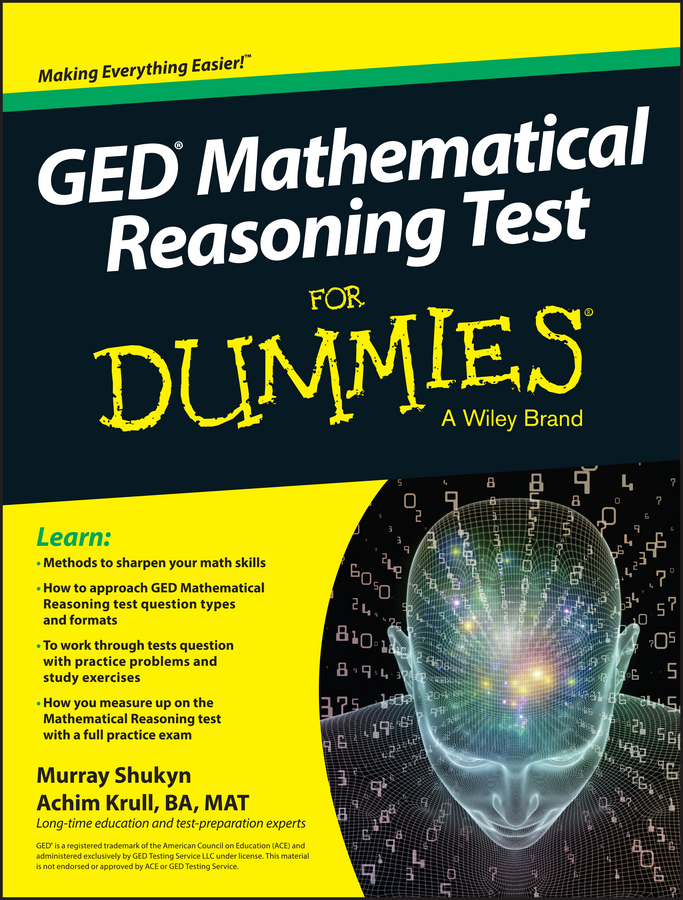Because you’re preparing in advance, you should walk into the testing room on PSAT/NMSQT day well acquainted, or even best friends, with the exam directions, especially for the math sections. Pressed for time, you don’t want to scan anything but the problems themselves on test day.

(Did you know that scan means “read carefully”? Many people confuse scan with skim, which is what you do when you zoom through reading material without thinking much.)

## Multiple-choice math questions

The multiple-choice math directions are fairly standard. You have to choose the correct answer from five possibilities. You’re allowed to use a calculator. The directions also state that all figures are on a plane (a two-dimensional, flat surface) unless otherwise stated. Diagrams are drawn to scale except when clearly labeled NOT drawn to scale.

However, the directions do more than simply tell you what to do. You don’t have to memorize math formulas before you take the PSAT/NMSQT, because tucked into the directions at the start of each math section is a little box of goodies: formulas for the area and circumference of a circle, the area of a rectangle and triangle, the volume of a cube and cylinder, and the angles of triangles.

Still, it’s best to know these formulas ahead of time so you don’t spend valuable time looking them up. Here’s what you see:

Notes:
• You may use a calculator.
• All numbers used in this exam are real numbers.
• All figures lie in a plane.
• You may assume that all figures are to scale unless the problem specifically indicates otherwise.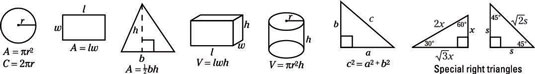There are 360 degrees of arc in a circle.
There are 180 degrees in a straight line.
There are 180 degrees in the sum of the interior angles of a triangle.

## Grid-ins

The last ten problems of the second math section are grid-ins. On your answer sheet you see ten boxes, each with four columns stacked with the numbers 0–9 and decimal points. Two of the columns have slanted fraction lines. Here’s a blank grid-in box: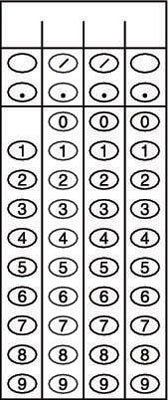For grid-in questions, first come up with an answer. Then follow these guidelines:

• Write your answer in the little squares at the top of the grid. Now you know what you have to bubble in. (In the context of the PSAT/NMSQT, to bubble means to “darken an oval with pencil.”) Remember that the little boxes are there to guide you. They are not scored.

• Darken the appropriate ovals. Bubble only one symbol or number per column.

• Start wherever you want. As long as your answer fits, don’t worry about which column you start in. If you want to bubble 59, for example, you can begin in the first, second, or third column. (You can’t begin in the fourth because you run out of room for the second digit, the 9.)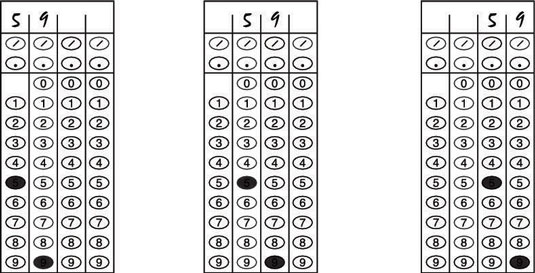• Don’t place a zero before a decimal point, unless it’s the last digit in a longer number. In other words, 50.3 is fine, but 0.53 isn’t. Go for .53 instead.

• Don’t round off a number unless it extends beyond the grid. When you do round off, be sure to use all four boxes. Suppose your solution is 7/123. You don’t have enough boxes for four digits and a slash, so you need a decimal. Your calculator tells you that the decimal equivalent of 7/123 is a very big bunch of numbers, beginning with .0569105.

You can round off to .057 (using all four boxes) but not to .06, because then you’d have one empty box. The PSAT/NMSQT counts that sort of rounding off as wrong because your answer is less specific than it should be.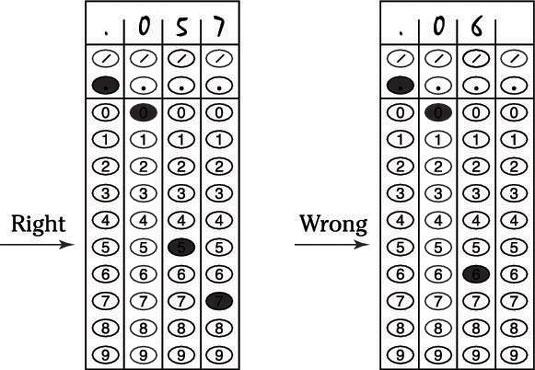• Don’t worry about negative numbers. No answer is negative; you have no bubble for a minus sign.

• If you come up with more than one solution, bubble only one answer. If your answer may be expressed as either a decimal or a fraction, choose one of those forms. Don’t try to squeeze in both.

Also, sometimes a PSAT/NMSQT math question has more than one possible solution. A prime number less than 10, for example, may be 2, 3, 5, or 7. Any of these answers would be graded as correct.

• Mixed numbers are a no-no. You can bubble in a fraction (5/8, for example), but you can’t record a mixed number. If you bubble in 5 ½, the computer reads your answer as 51/2 If you end up with a mixed number (such as 5 ½), record it as 11/2 (an improper fraction) or as a decimal (5.5).

The multiple-choice and grid-in directions aren’t exactly rocket science, but they are important. When you take the practice exams in this book, check your gridding as well as your mathematical ability, because both are necessary for success.